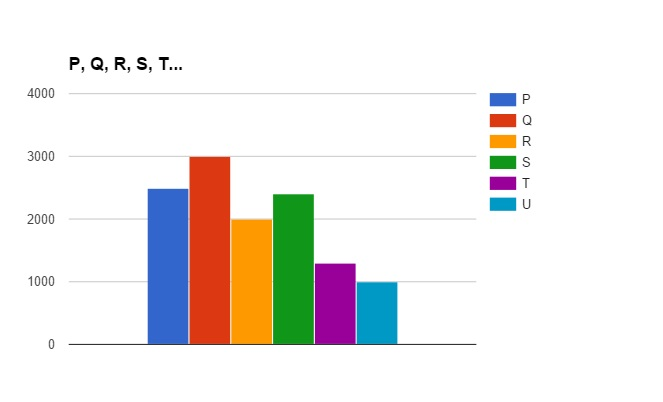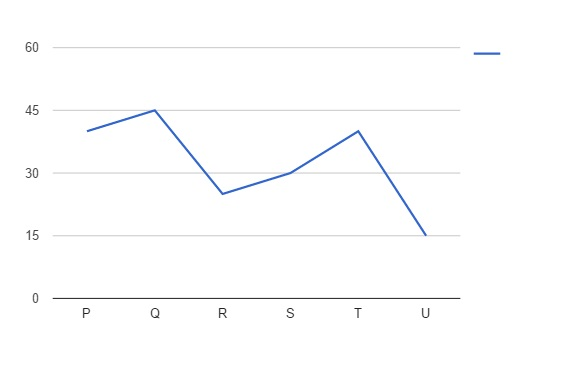## RBI Assistant Data Analysis Test 11

Instructions

Study the graphs carefully to answer the question that follows:
Total number of children in 6 different schools and the percentage of girls in themQ 1

What is the total percentage of boys in schools R and U together ? (rounded off to two digits after decimal)

Q 2

What is the total number of boys in School T ?

Q 3

The total number of students in school R is approximately what per cent of the total number of students in School S?

Q 4

What is the average number of boys in schools P and Q together ?

Q 5

What is the ratio of the number of girls in school P to the number of girls in school Q ?